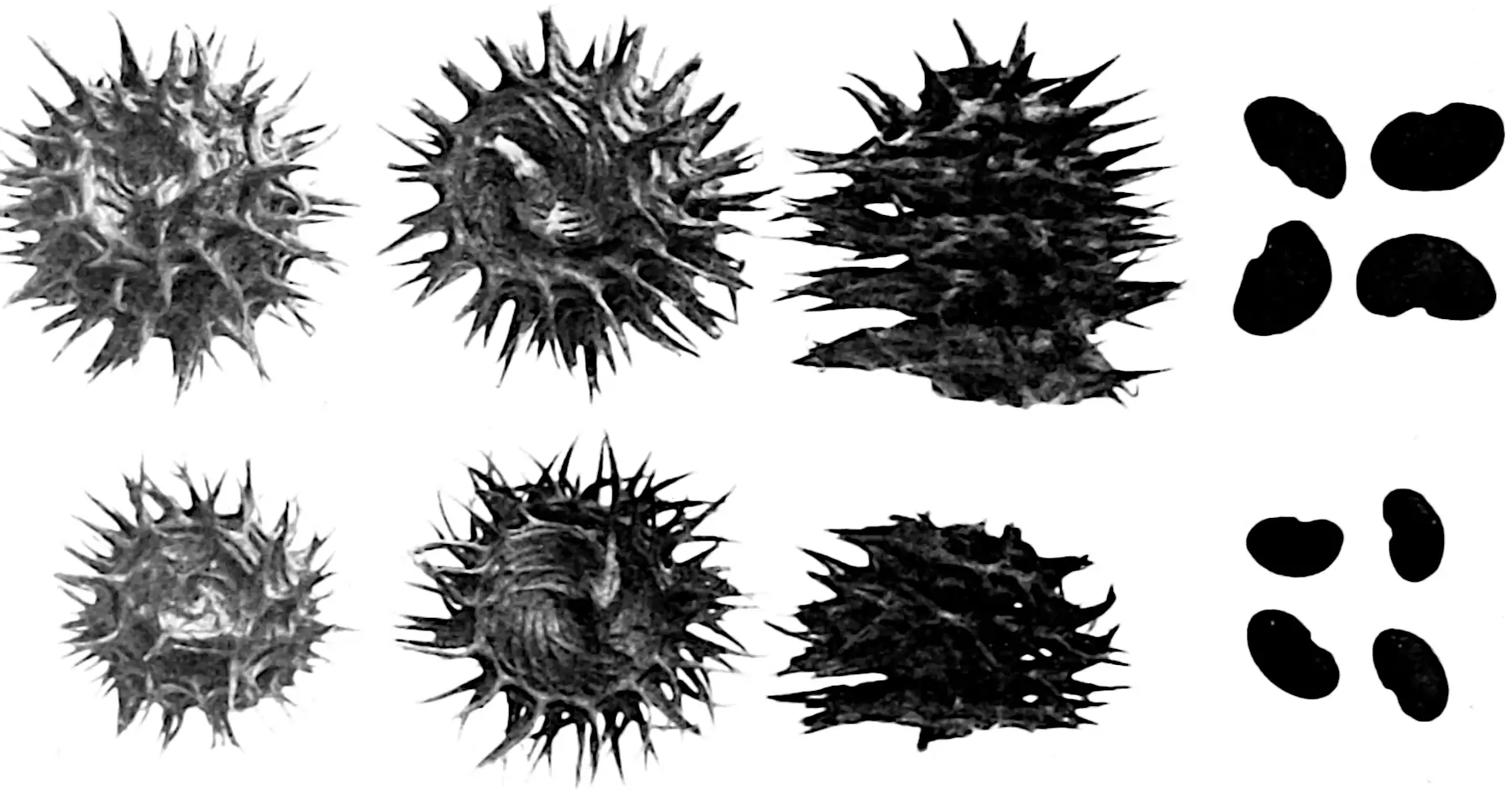# Lévy Gamma processes

$\renewcommand{\var}{\operatorname{Var}} \renewcommand{\corr}{\operatorname{Corr}} \renewcommand{\dd}{\mathrm{d}} \renewcommand{\bb}{\mathbb{#1}} \renewcommand{\vv}{\boldsymbol{#1}} \renewcommand{\rv}{\mathsf{#1}} \renewcommand{\vrv}{\vv{\rv{#1}}} \renewcommand{\disteq}{\stackrel{d}{=}} \renewcommand{\gvn}{\mid} \renewcommand{\Ex}{\mathbb{E}} \renewcommand{\Pr}{\mathbb{P}}$

Processes with Gamma marginals. Usually when we discuss Gamma processes we mean Gamma-Lévy processes. Such processes have independent Gamma increments, much like a Wiener process has independent Gaussian increments and a Poisson process has independent Poisson increments. Gamma processes provide the classic subordinator models, i.e. non-decreasing Lévy processes.

There are other processes with Gamma marginals.Gamma processes are a natural model for spiky things

OK but if a process’s marginals are “Gamma-distributed”, what does that even mean? First, go and read about Gamma distributions. THEN go and read about Beta and Dirichlet distributions. We need both. And especially the Gamma-Dirichlet algebra.

For more, see the Gamma-Beta notebook.

## The Lévy-Gamma process

Every divisible distribution induces an associated Lévy process by a standard procedure This works on the Gamma process too.

Ground zero for treating these processes specifically appears to be Ferguson and Klass (1972), and then the weaponisation of these processes to construct the Dirichlet process prior occurs in Ferguson (1974). Tutorial introductions to Gamma(-Lévy) processes can be found in . Existence proofs etc are deferred to those sources. You could also see Wikipedia, although that article was not particularly helpful for me.

The univariate Lévy-Gamma process $$\{\rv{g}(t;\alpha,\lambda)\}_t$$ is an independent-increment process, with time index $$t$$ and parameters by $$\alpha, \lambda.$$ We assume it is started at $$\rv{g}(0)=0$$.

The marginal density $$g(x;t,\alpha, \lambda )$$ of the process at time $$t$$ is a Gamma RV, specifically, $g(x;t, \alpha, \lambda) =\frac{ \lambda^{\alpha t} } { \Gamma (\alpha t) } x^{\alpha t\,-\,1}e^{-\lambda x}, x\geq 0.$ We can think of the Gamma distribution as the distribution at time 1 of a Gamma process.

That is, $$\rv{g}(t) \sim \operatorname{Gamma}(\alpha(t_{i+1}-t_{i}), \lambda)$$. which corresponds to increments per unit time in terms of $$\bb E(\rv{g}(1))=\alpha/\lambda$$ and $$\var(\rv{g}(1))=\alpha/\lambda^2.$$

Aside: Note that the useful special case that $$\alpha t=1,$$ then we have that $$\rv{g}(t;1,\lambda )\sim \operatorname{Exp}(\lambda).$$

The increment distirbution leads to a method for simulating a path of a Gamma process at a sequence of increasing times, $$\{t_1, t_2, t_3, \dots, t_L\}.$$ Given $$\rv{g}(t_1;\alpha, \lambda),$$ we know that the increments are distributed as independent variates $$\rv{g}_i:=\rv{g}(t_{i+1})-\rv{g}(t_{i})\sim \operatorname{Gamma}(\alpha(t_{i+1}-t_{i}), \lambda)$$. Presuming we may simulate from the Gamma distribution, it follows that $\rv{g}(t_i)=\sum_{j < i}\left( \rv{g}(t_{i+1})-\rv{g}(t_{i})\right)=\sum_{j < i} \rv{g}_j.$

### Lévy characterisation

For arguments $$x, t>0$$ and parameters $$\alpha, \lambda>0,$$ we have the increment density as simply a Gamma density:

$p_{X}(t, x)=\frac{\lambda^{\alpha t} x^{\alpha t-1} \mathrm{e}^{-x \lambda}}{ \Gamma(\alpha t)},$

This gives us a spectrally positive Lévy measure $\pi_{\rv{x}}(x)=\frac{\alpha}{x} \mathrm{e}^{-\lambda x}$ and Laplace exponent $\Phi_{\rv{x}}(z)=\alpha \ln (1+ z/\lambda), z \geq 0.$

That is, the Poisson rate, with respect to time $$t$$ of jumps whose size is in the range $$[x, x+dx)$$, is $$\pi(x)dx.$$ We think of this as an infinite superposition of Poisson processes driving different sized jumps, where the jumps are mostly tiny. This is how I think about Lévy process theory, at least.

## Gamma bridge

A useful associated process. Consider a univariate Gamma-Lévy process, $$\rv{g}(t)$$ with $$\rv{g}(0)=0.$$ The Gamma bridge, analogous to the Brownian bridge, is that process conditionalised upon attaining a fixed the value $$S=\rv{g}(1)$$ at terminal time $$1.$$ We write $$\rv{g}_{S}:=\{\rv{g}(t)\mid \rv{g}(1)=S\}_{0< t < 1}$$ for the paths of this process.

We can simulate from the Gamma bridge easily. Given the increments of the process are independent, if we have a Gamma process $$\rv{g}$$ on the index set $$[0,1]$$ such that $$\rv{g}(1)=S$$, then we can simulate from the bridge paths which connect these points at intermediate time $$t,\, 0<t<1$$ by recalling that we have known distributions for the increments; in particular $$\rv{g}(t)\sim\operatorname{Gamma}(\alpha, \lambda)$$ and $$\rv{g}(1)-\rv{g}(t)\sim\operatorname{Gamma}(\alpha (1-t), \lambda)$$ and these increments, as increments over disjoints sets, are themselves independent. Then, by the Beta thinning, $\frac{\rv{g}(t)}{\rv{g}(1)}\sim\operatorname{Beta}(\alpha t, \alpha(1-t))$ independent of $$\rv{g}(1).$$ We can therefore sample from a path of the bridge $$\rv{g}_{S}(t)$$ for some $$t< 1$$ by simulating $$\rv{g}_{S}(t)=B S,$$ where $$B\sim \operatorname{Beta}(\alpha (t),\alpha (1-t)).$$

For more on that theme, see Barndorff-Nielsen, Pedersen, and Sato (2001), Émery and Yor (2004) or Yor (2007).

## Completely random measures

Random probability distributions induced by using Gamma-Lévy processes as a CDF. I laboriously reinvented these, bemused that no one seemed to use them, before discovering that they are called “completely random measures” and they are in fact pretty common. So that was a fun exercise.

## Time-warped Lévy-Gamma process

Çinlar (1980) walks us through the mechanics of (deterministically) time-warping Gamma processes, which ends up being not too unpleasant. Predictable stochastic time-warps look like they should be OK. See N. Singpurwalla (1997) for an application. Why bother? Linear superpositions of Gamma processes can be hard work, and sometime the generalisation from time-warping can come out nicer supposedly. 🏗

### No comments yet. Why not leave one?

GitHub-flavored Markdown & a sane subset of HTML is supported.